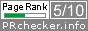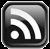# LEARN MS-ACCESS TIPS AND TRICKS

Learn Microsoft Access Advanced Programming Techniques, Tips and Tricks.

### Introduction.

Before taking up the above subject let us look at some fundamentals on variables for the benefit of novices.

When we define a variable in VBA or in any other programming language the computer reserves some memory location and allocates some memory cells (the number of cells allocated depends on the declared variable type, like Integer, Double, String, etc.) to store values passed to it.

In layman's analogy, we can imagine a variable as a box with the name 'Apple' or whatever name we give to the box and uses that name to pick the value stored in it.  Assume that you have put 5 in the Apple box.  We can give these apples to someone in two ways.

1. Make copies of the apples (the number) from the box ourselves and put it into another box and pass it.  The target box's name will be different.  The recipient of the new box can work with his copy of the apples, like add more apples into his box or remove some of them, etc.  There will not be any change in the first box contents.
2. We can tell the other person (or Function), which area of the room (location) you have kept the original box of apples, get the box contents from there, and work with it.  In this room (or within the function body) there may be other boxes (Variables) with different names and contents.

### Making Copies of Original Values.

In the first method explained above, unlike the physical box, you can make copies of the original value and store them into different Variables.  The original value will not change in the first Variable.  The Function that gets the new Variable with the copy has no access to the first Variable.  He can do whatever he wants to do with the copy he has.

In the second case you can tell the location of the Apple_Box1 to the other Function so that it can go there and find it's contents and do whatever the Function wants to do with them (add, subtract, multiply, etc. or use it as part of other calculations) or whatever operations you would like to do with them.

To prove the first point above let us take a closer look at the next two example functions Test_1A() (the calling function) and Test_1B() (the called function with the copy of the value).

#### Method-1 Examples:

```Public Function Test_1A()

'Define two variables(boxes)to hold values of
'Long Integer type
Dim Apple_Box1 As Long, ApplesReceived As Long

'Put an initial value of 10

'into the variable

Apple_Box1 = 10

'sending a copy of Apple_Box1 Value to Apple_Box2

'Whatever value changes happened in Apple_Box2 in Test_1B()

'call funcction Test_1B() with the value
'Display the result in MsgBox.
MsgBox "Apple_Box1: " & Apple_Box1 & vbLf & "Apple_Box2 contents: " & ApplesReceived

End Function```

In the above program we are defining two Variables Apple_Box1 and ApplesReceived both to store Long Integer type values. Here, we are not going to bother about what is Long Integer or Short Integer and their range of values that we can store in them, etc.

Next line Apple_Box1 = 10, value 10 is stored in Apple_Box1.

The next three lines are remarks explaining what we are doing in the next line.

We are calling Test_1B() Function and passing the value of Apple_Box1 to the function to do something with the value received in a Variable (ByVal Apple_Box2).  The ByVal before Apple_Box2 given in Test_1B() function states that take a copy of the value from Apple_Box1.  The ‘As Long’ appearing after the closing parenthesis indicates that the second function does some calculations with the received value and returns the result to the first function.  The new value received is stored in ApplesReceived Variable.

Next line MsgBox() function displays the Apple_Box1 contents and the result value (after modification done to the original copy of value) received from Test_1B() function.

### The Test_1B Function.

```Public Function Test_1B(ByVal Apple_Box2 As Long) As Long

'Take Note of the Key-Word 'ByVal' (says take a Copy of the Passed Value)
'Return the value back into the first function Test_1A
'After adding 15 to the original value of 10
'copied into Apple_Box2

Test_1B = Apple_Box2 + 15

End Function
```

There is only one executable statement in the above function.  Immediately after the function definition four lines of remarks indicating what happens in the function.

We will take a closer look at the next line of the statement. This statement has two parts – the first part appears left of the = sign and the second part is on the right side of the equal sign.

In this expression, the left side of the equal sign will be a Variable or a Function name.  By now you will be asking yourself as to why a function name there. That will be explained in a moment.

The computer always evaluates the expression given at the right side of the equal sign first and arrives at a single value and moves that result in the Variable given at the left side of the equal sign.  Any existing value in the variable will be lost. You can write that expression in two lines to arrive at the same result as below:

Apple_Box2 = Apple_Box2 + 15

Test_1B = Apple_Box2

In the first expression, you can see that we have used the Apple_Box2 variable to the left side and right side of the = sign. As I said earlier the expression at the right side of the equal sign is evaluated first. So it takes the existing value of 10 from Apple_Box2 for calculations and Adds 15 to it, arriving at the single result value of 25, and moves that value into Variable Apple_Box2, replacing earlier value 10.

If the Function name (the function name given at the first line of Code) appears at the left side of equal sign then the meaning of the statement is that the final result of the expression must be returned to the Calling Function.  Here, the Function Name acts as a Variable with the Data Type (As Long) specified immediately after the closing brackets on the first line.

This function name appears on the calling statement in the calling function to the right side of the = sign and a Variable Name on the left side of the = sign that saves the received result value (ApplesReceived = Test_1B(Apple_Box1).

#### Method-2 Examples:

In this method, we have defined only one variable Apple_Box1 as Long Integer Type.  In the next line, the Variable is loaded with an initial value of 10.  The next two lines are remarks explaining what is happening in the next line that calls the second function Test_1D().

Compare this statement with the statement that calls Test_1B.  Unlike the statement that calls Test_1B() the Function Name Test_1D and the function parameter, Variable Apple_Box1 only appear here.  The opening and closing brackets are omitted from the function name. The parameter variable is the second item.  Test_1D() function is not returning any value back into the calling function Test_1C.  Therefore we don't need to write this line of code in the form of an expression as we did in Test_1A Function.  But, you cannot write the statement as:

Test_1D(Apple_Box1).,

Once we use the parenthesis (normally used with the function name) around the parameter variable then VBA assumes that some value is returned from the called function and you are forced to write it like we did it in Function Test_1A:

```x = Test_1D(Apple_Box1) 'Expression
'OR use Call statement
Call Test_1D(Apple_Box1)
```

There will not be any value in the variable x because no value is returned from the called function.

If you feel comfortable with this method then you may do so. You will be defining one more variable for this purpose and your program takes up more memory.

The usage of the Call statement requires the parenthesis around the parameter variable. If no parameters to pass to the called function even then you should use the opening and closing parenthesis at the end of Function Name like Call Test_1D().

When control returns from Test_1D() the next line displays the changed value in Apple_Box1 Variable.

```Public Function Test_1C()
Dim Apple_Box1 As Long

'put 10 into Variable
Apple_Box1 = 10

'here Test_1D function takes the

Test_1D Apple_Box1 'compare this statement with Test_1A function

MsgBox "Apple_Box1: " & Apple_Box1 & vbLf & "Apple_Box2 contents: " & ApplesReceived

End Function

```

Test_1D() function takes the location address of the parameter variable passed to it. It works directly with the value stored in the variable Apple_Box1's own location.

```Public Function Test_1D(ByRef Apple_Box2 As Long)

Apple_Box2 = Apple_Box2 + 15

End Function

```

Test_1D() takes the location address (this is the memory location number of the variable) of variable Apple_Box1 into variable Apple_Box2, defined in the parameter area of Test_1D() function.

Now, look at the expression:

Apple_Box2 = Apple_Box2 + 15

Apple_Box2 contains the location address, not the contents of the Apple_Box1 variable. But, no need to make any change in the expression to do any kind of calculations.  The computer uses the location address to pick the value from there and use the value in calculations.

Even though we have used ByRef to the Parameter Variable, to accept the location address of the variable passed to it (always a number irrespective of different variable types), the rest of the parameter definition is like any other variable with variable Type (as Long) specification.  Compare Test_1D() function definition with the Test_1B().  Test_1D doesn't have the As Long at the end of the line because it is not returning any value from the function but it changes the original value at its location directly.

You may omit the usage ByRef from the Function declaration. By default, VBA assumes that the Function Parameter declaration is ByRef (By Reference) if you have not explicitly defined the parameter as ByVal like:

`Public Function Test_1D(Apple_Box2 As Long)`

Each Variable Type, like Byte, Integer, Long (integer), Double, String, etc. gets allocated with enough memory cells, besides its own address, to hold their maximum range of values. This is different among Programming Languages: VBA, C, C++, C#, etc.

### In Conclusion.

We don't have to bother about going too deep into all those things but it does no harm to have a basic understanding of them.

If you get in touch with the C language and its variants, like the examples given above, you need to deal with these things, sooner or later.

Our discussion here was on a single variable and it's value. How we can work with an Array of Variables and Values. We will explore its methods in the coming weeks.

Share:

#### 1 comment:

1.I think it'd be better to have the date of the post instead of the time
a.p.r. pillai 7:30 AM No comments

Comments subject to moderation before publishing.

## TranslateSubscribe in a readerYour email address:

Delivered by FeedBurner